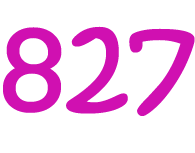Pull Down & Release to Refresh

# Definition of NonaryNonary numbers are made up only of the digits $\{0,1,2,3,4,5,6,7,8\}$. Each place in a nonary number corresponds to a power of $9$.

Because there are only $9$ different possible digits in a nonary number, we also call them Base $9$ numbers.

For example, the nonary number $827$ is equal to the decimal number $8 \times 9^2 + 2 \times 9^1 + 7 \times 9^0 = 8 \times 81 + 2 \times 9 + 7= 673$.

Some other examples of nonary numbers are:

• $88$, which corresponds to the decimal number $80$.
• $100$, which corresponds to the decimal number $81$.
• $105$, which corresponds to the decimal number $86$.

### Description

The aim of this dictionary is to provide definitions to common mathematical terms. Students learn a new math skill every week at school, sometimes just before they start a new skill, if they want to look at what a specific term means, this is where this dictionary will become handy and a go-to guide for a student.

### Audience

Year 1 to Year 12 students

### Learning Objectives

Learn common math terms starting with letter N

Author: Subject Coach
You must be logged in as Student to ask a Question.